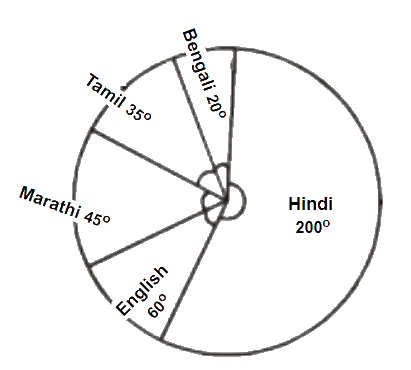# Ex.5.2 Q5 Data Handling Solutions - NCERT Maths Class 8

Go back to  'Ex.5.2'

## Question

The number of students in a hostel, speaking different languages is given below. Display the data in a pie chart.

 Language Hindi English Marathi Tamil Bengali Total No. of students $$40$$ $$12$$ $$9$$ $$7$$ $$4$$ $$72$$

## Text Solution

What is known?

Distribution of the students with respect to the language they speak.

What is unknown?

Pie chart of the given information.

Reasoning:

A circle graph or pie chart shows the relationship between a whole and its parts.Sum of the total angles in a pie chart is equal to $$360^{\circ}.$$

Steps:

The central angle for each language is calculated as below

 Language No. of students In fraction Central Angle Hindi $$40$$ \begin{align}\frac{{40}}{{72}} = \frac{5}{9}\end{align} \begin{align}\frac{5}{9} \times 360^\circ = 200^\circ \end{align} English $$12$$ \begin{align}\frac{{12}}{{72}} = \frac{1}{6}\end{align} \begin{align}\frac{1}{6} \times {360^{\circ}} = 60^\circ\end{align} Marathi $$9$$ \begin{align}\frac{9}{{72}} = \frac{1}{8}\end{align} \begin{align}\frac{1}{8} \times 360^\circ = 45^\circ\end{align} Tamil $$7$$ \begin{align}\frac{7}{{72}} = \frac{7}{{72}}\end{align} \begin{align}\frac{7}{{72}} \times {360^\circ} = {35^\circ}\end{align} Bengali $$4$$ \begin{align}\frac{4}{{72}} = \frac{1}{{18}}\end{align} \begin{align}\frac{1}{{18}} \times {360^\circ} = {20^\circ}\end{align} Total $$72$$

Pie chart for above data is drawn as below:Learn from the best math teachers and top your exams

• Live one on one classroom and doubt clearing
• Practice worksheets in and after class for conceptual clarity
• Personalized curriculum to keep up with school